# How to set the spacing between subplots in Matplotlib in Python?

In this article, we will see how to set the spacing between subplots in Matplotlib in Python. Let’s discuss some concepts :

• Matplotlib : Matplotlib is an amazing visualization library in Python for 2D plots of arrays. Matplotlib is a multi-platform data visualization library built on NumPy arrays and designed to work with the broader SciPy stack. It was introduced by John Hunter in the year 2002.
• Subplots : The subplots() function in pyplot module of matplotlib library is used to create a figure and a set of subplots. Subplots are required when we want to show two or more plots in same figure.

Here, first we will see why setting of space is required.

## Python3

 `# importing packages` `import` `numpy as np` `import` `matplotlib.pyplot as plt`   `# create data` `x``=``np.array([``1``, ``2``, ``3``, ``4``, ``5``])`   `# making subplots` `fig, ax ``=` `plt.subplots(``2``, ``2``)`   `# set data with subplots and plot` `ax[``0``, ``0``].plot(x, x)` `ax[``0``, ``1``].plot(x, x``*``2``)` `ax[``1``, ``0``].plot(x, x``*``x)` `ax[``1``, ``1``].plot(x, x``*``x``*``x)` `plt.show()`

Output: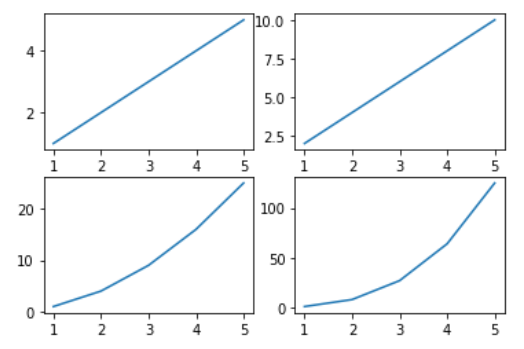Too much congested and very confusing

## Set the spacing between subplots

As, we can see that the above figure axes values are too congested and very confusing. To solve this problem we need to set the spacing between subplots.

### Steps Needed

1. Import Libraries
3. Make subplot
4. Plot subplot
5. Set spacing between subplots.

## Using tight_layout() method to set the spacing between subplots

The tight_layout() method automatically maintains the proper space between subplots.

## Python3

 `# set the spacing between subplots` `fig.tight_layout()` `plt.show()`

Output: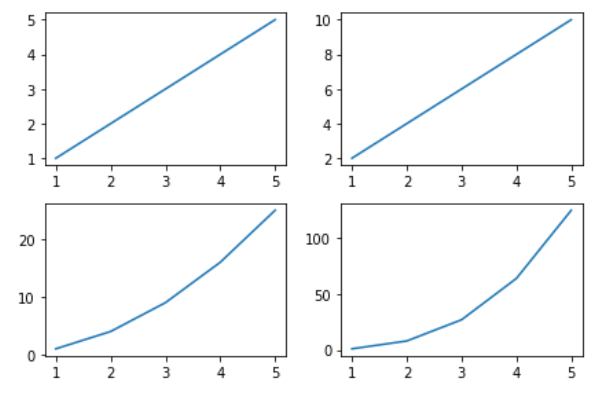set the spacing between subplots in Matplotlib

## Python3

 `# using padding` `fig.tight_layout(pad``=``5.0``)`   `plt.show()`

Output: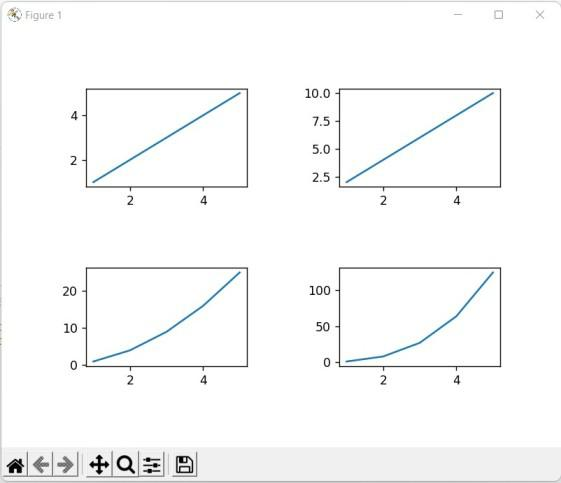set the spacing between subplots in Matplotlib

## Using subplots_adjust() method to set the spacing between subplots

We can use the plt.subplots_adjust() method to change the space between Matplotlib subplots. The parameters wspace and hspace specify the space reserved between Matplotlib subplots. They are the fractions of axis width and height, respectively. And the parameters left, right, top and bottom parameters specify four sides of the subplots’ positions. They are the fractions of the width and height of the figure.

## Python3

 `# set the spacing between subplots` `plt.subplots_adjust(left``=``0.1``,` `                    ``bottom``=``0.1``, ` `                    ``right``=``0.9``, ` `                    ``top``=``0.9``, ` `                    ``wspace``=``0.4``, ` `                    ``hspace``=``0.4``)` `plt.show()`

Output: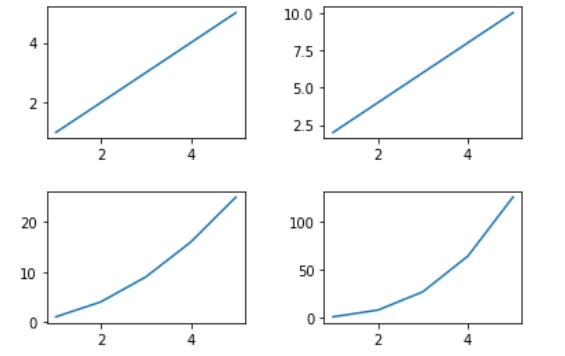## Using subplots_tool() method to set the spacing between subplots

This method launches a subplot tool window for a figure. It provides an interactive method for the user to drag the bar in the subplot_tool to change the subplots’ layout.

## Python3

 `# set the spacing between subplots` `plt.subplot_tool()` `plt.show()`

Output: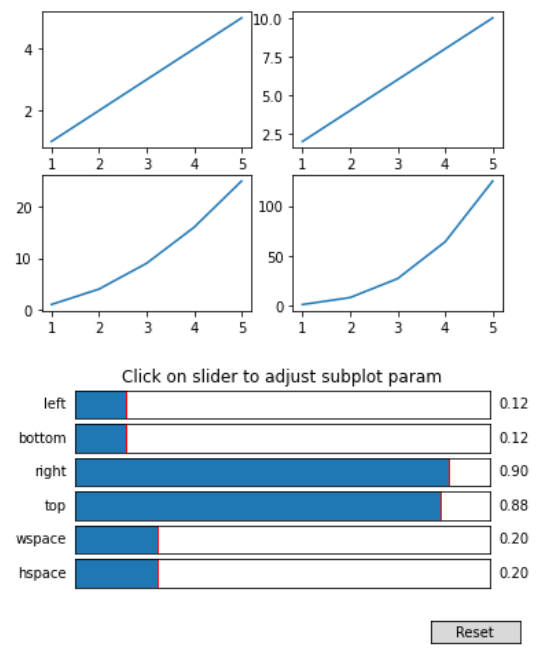## Using constrained_layout() to set the spacing between subplots

Here we are using the constrained_layout=True to set the spacing between the subplots in Matplotlib.

## Python3

 `# making subplots with constrained_layout=True` `fig, ax ``=` `plt.subplots(``2``, ``2``, ` `                       ``constrained_layout ``=` `True``)`   `# set data with subplots and plot` `ax[``0``, ``0``].plot(x, x)` `ax[``0``, ``1``].plot(x, x``*``2``)` `ax[``1``, ``0``].plot(x, x``*``x)` `ax[``1``, ``1``].plot(x, x``*``x``*``x)` `plt.show()`

Output: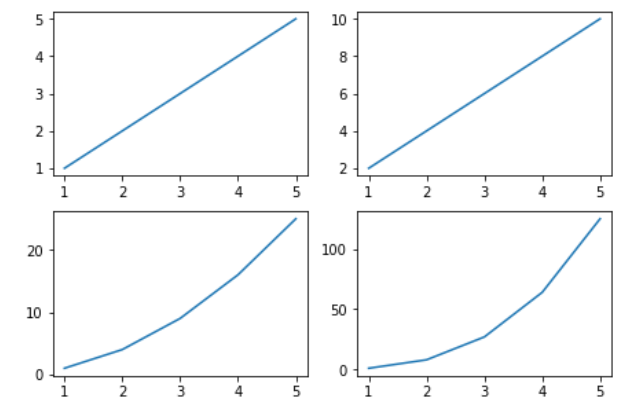Whether you're preparing for your first job interview or aiming to upskill in this ever-evolving tech landscape, GeeksforGeeks Courses are your key to success. We provide top-quality content at affordable prices, all geared towards accelerating your growth in a time-bound manner. Join the millions we've already empowered, and we're here to do the same for you. Don't miss out - check it out now!

Previous
Next